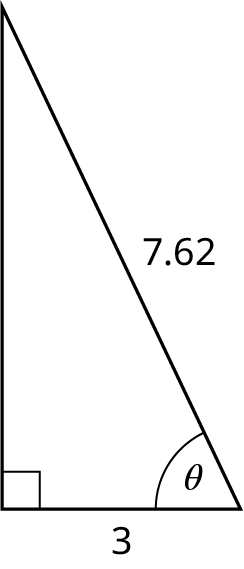Contemporary Mathematics

# Chapter Test

### Chapter Test

Use the given figure for the following exercises.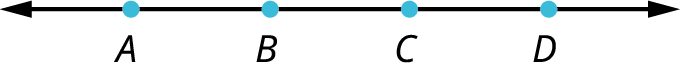1.
Find \overleftrightarrow {AD} \cap \overline {CD}.
2.
Find {\overline {AB}} \cup {\overline {BC}}.
3.
Use the given figure to find the angle measurements.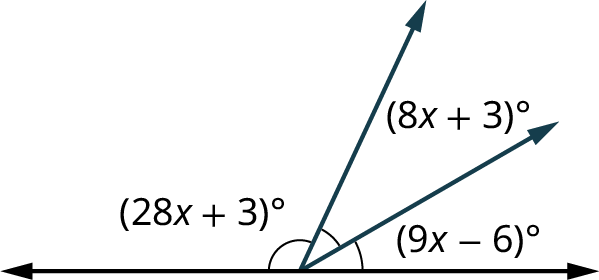4.
Given that {l_1} and {l_2} are parallel lines, solve the angle measurements for all the angles in the given figure.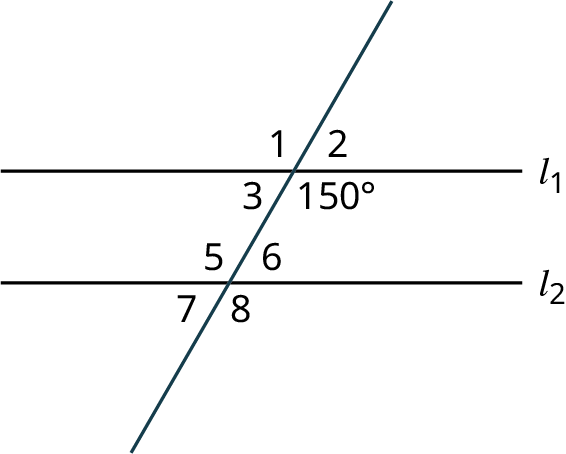5.
Find the angle measurements in the given figure.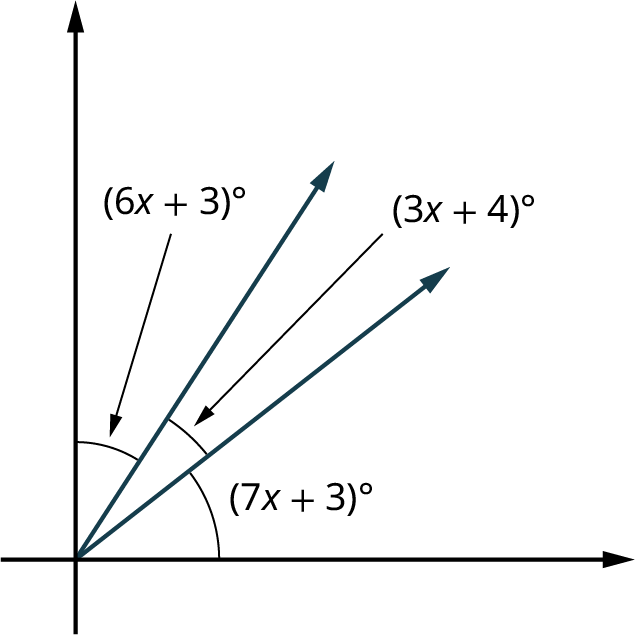6.
The two triangles shown are congruent by what theorem?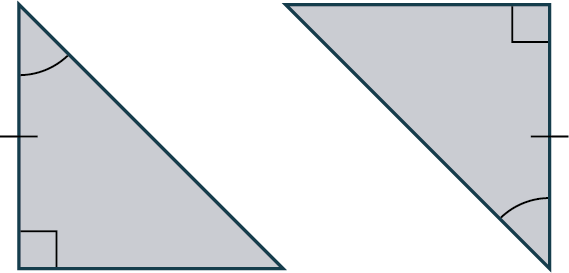7.
Find the sum of the interior angles of a regular heptagon.
8.
Determine the scaling factor between these two similar triangles.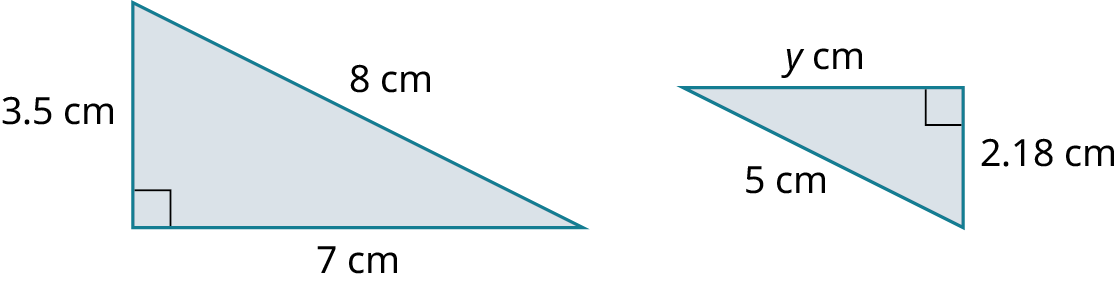9.
Find the measure of the missing angles in the figure shown.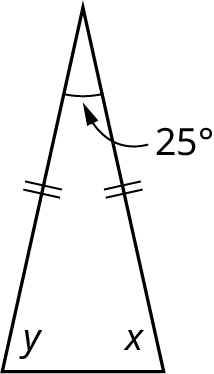10.
Calculate the perimeter of a regular octagon with a side length of 5 cm.
11.
Find the measurement of an interior angle of a regular heptagon.
12.
Find the sum of the interior angles of a regular pentagon.
13.
Find the measure of an exterior angle of a regular pentagon.
14.
Find the circumference of the circle with a radius of 3.5 cm.
15.
What are the four transformations that are used to produce tessellations?
16.
Find the surface area of the triangular prism shown.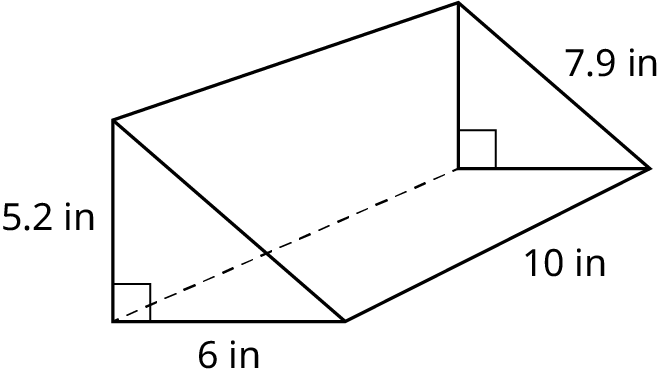17.
Find the volume of the right cylinder in the given figure.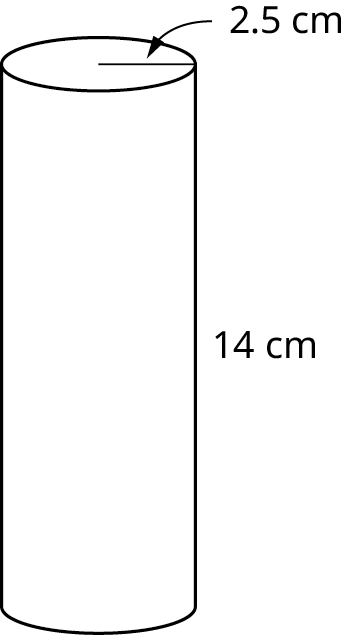18.
Find the missing length in the given figure.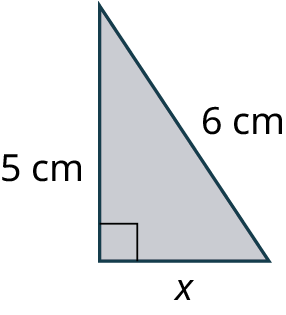19.
Find the missing length in the given figure.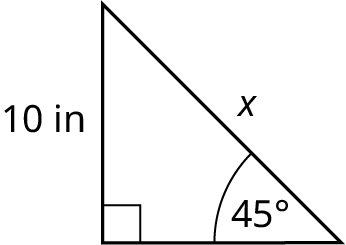20.
Find the length of side b in the given figure.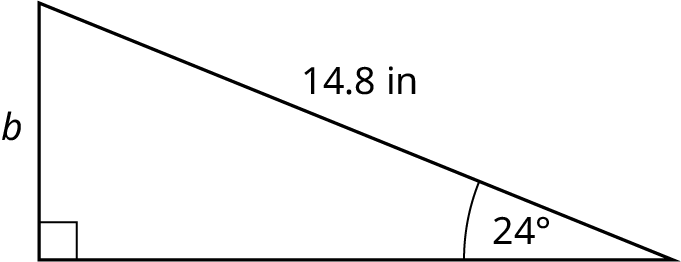21.
Find the length of side a in the given figure.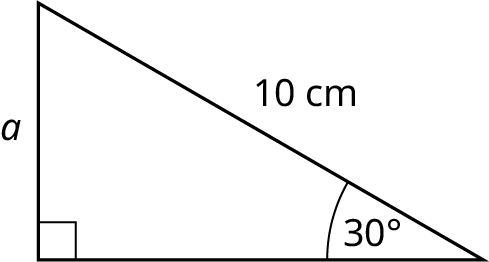22.
Find the measure of \theta in the given figure.